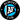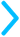## Slope Intercept Form

There are two main steps to getting the slope-intercept form of a line - and they're in the name. We need to first find the slope, and then find the y-intercept.

You can read the steps below, or you can scroll down to our calculator (you know you want to)

and try it out yourself 😎.

#### Slope-Intercept Formula

The slope-intercept form of a line looks like this:$\textcolor{#00bbff}{y = mx +b}$$m$ stands for the slope and $b$ stands for the y-intercept of the line. The steps to write this equation are below.
1. First, find two points on the line. Let's call them Point 1 with coordinates $\textcolor{#F18F01}{(x_1,y_1)}$ and Point 2 with coordinates $\textcolor{#A14DA0}{(x_2,y_2)}$.
2. Once we have our two points, we'll find the slope. Divide the change in $y$ by the change in $x$:$m = \text{slope} = \frac{\textcolor{#F18F01}{y_1} - \textcolor{#A14DA0}{y_2}}{\textcolor{#F18F01}{x_1} - \textcolor{#A14DA0}{x_2}}$
Notice the color coding - make sure that the first $x$ and $y$ values in the subtractions are from the same point.
3. Now, let's pretend that the value we got for $m$ is $2$. We can plug that back into our equation, so now we have$y = 2x + b$
To get $b$, we just plug in one of our points -$\textcolor{#F18F01}{y_1} = 2(\textcolor{#F18F01}{x_1}) + b$
- and solve for $b$.
If this is all overwhelming, no sweat, try it step-by-step below👇🏻👇🏽👇🏿.

## Slope-Intercept Form Calculator

### Point 1

$x_1=$
$y_1=$

### Point 2

$x_2=$
$y_2=$